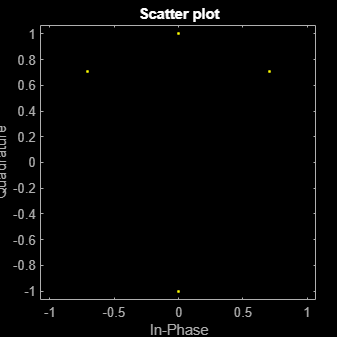# comm.GeneralQAMTCMModulator

Convolutionally encode binary data and map using arbitrary QAM constellation

## Description

The `GeneralQAMTCMModulator` object implements trellis-coded modulation (TCM) by convolutionally encoding the binary input signal. The object then maps the result to an arbitrary signal constellation. The Signal constellation property lists the signal constellation points in set-partitioned order.

To modulate a signal using a trellis-coded, general quadrature amplitude modulator:

1. Define and set up your general QAM TCM modulator object. See Construction.

2. Call `step` to modulate a signal according to the properties of `comm.GeneralQAMTCMModulator`. The behavior of `step` is specific to each object in the toolbox.

Note

Starting in R2016b, instead of using the `step` method to perform the operation defined by the System object™, you can call the object with arguments, as if it were a function. For example, ```y = step(obj,x)``` and `y = obj(x)` perform equivalent operations.

## Construction

`H = comm.GeneralQAMTCMModulator` creates a trellis-coded, general quadrature amplitude (QAM TCM) modulator System object, `H`. This object convolutionally encodes a binary input signal and maps the result using QAM modulation with a signal constellation specified in the Constellation property.

`H = comm.GeneralQAMTCMModulator(Name,Value)` creates a general QAM TCM modulator System object, `H`, with each specified property set to the specified value. You can specify additional name-value pair arguments in any order as (`Name1`,`Value1`,...,`NameN`,`ValueN`).

`H = comm.GeneralQAMTCMModulator(TRELLIS,Name,Value)` creates a general QAM TCM modulator System object, `H`. This object has the `TrellisStructure` property set to `TRELLIS`, and the other specified properties set to the specified values.

## Properties

 `TrellisStructure` Trellis structure of convolutional code Specify trellis as a MATLAB® structure that contains the trellis description of the convolutional code. Use the `istrellis` function to check if a structure is a valid trellis structure. The default is the result of `poly2trellis`(`[1 3]`, ```[1 0 0; 0 5 2]```). `TerminationMethod` Termination method of encoded frame Specify the termination method as one of `Continuous` | `Truncated` | `Terminated`. The default is `Continuous`. When you set this property to `Continuous`, the object retains the encoder states at the end of each input vector for use with the next input vector. When you set this property to `Truncated`, the object treats each input vector independently. The encoder is reset to the all-zeros state at the start of each input vector. When you set this property to `Terminated`, the object treats each input vector independently. For each input vector, the object uses extra bits to set the encoder to the all-zeros state at the end of the vector. For a rate K/N code, the `step` method outputs the vector with length $y=N×\left(L+S\right)}{K}$, where S = constraintLength–1. In the case of multiple constraint lengths, S = sum(constraintLength(i)–1)). L represents the length of the input to the `step` method. `ResetInputPort` Enable modulator reset input Set this property to `true` to enable an additional input to the `step` method. The default is `false`. When this additional reset input is a nonzero value, the internal states of the encoder reset to their initial conditions. This property applies when you set the `TerminationMethod` property to `Continuous`. `Constellation` Signal constellation Specify a double- or single-precision complex vector that lists the points in the signal constellation that were used to map the convolutionally encoded data. You must specify the constellation in set-partitioned order. See documentation for the General TCM Encoder block for more information on set-partitioned order. The length of the constellation vector must equal the number of possible input symbols to the convolutional decoder of the general QAM TCM demodulator object. This corresponds to 2N for a rate K/N convolutional code. The default corresponds to a set-partitioned order for the points of an 8-PSK signal constellation. This value is expressed exp($2×\pi ×j×\left[\begin{array}{cccccccc}0& 4& 2& 6& 1& 5& 3& 7\end{array}\right]}{8}$). `OutputDataType` Data type of output Specify the output data type as one of `double` | `single`. The default is `double`.

## Methods

 step Convolutionally encode binary data and map using arbitrary QAM constellation
Common to All System Objects
`release`

Allow System object property value changes

`reset`

Reset internal states of System object

## Examples

collapse all

Modulate data using QAM TCM modulation with an arbitrary 4-point constellation. Display a scatter plot of the modulated data.

Create binary data.

`data = randi([0 1],1000,1);`

Use the trellis structure with generating polynomial [171 133] and 4-point arbitrary constellation { ${e}^{j\pi /4}$, ${e}^{j\pi /2}$, ${e}^{j3\pi /4}$, ${e}^{j3\pi /2}$ } to perform QAM TCM modulation.

```t = poly2trellis(7,[171 133]); hMod = comm.GeneralQAMTCMModulator(t,... 'Constellation',exp(pi*1i*[1 2 3 6]/4));```

Modulate and plot the data.

```modData = step(hMod,data); scatterplot(modData);```## Algorithms

This object implements the algorithm, inputs, and outputs described on the General TCM Encoder block reference page. The object properties correspond to the block parameters.